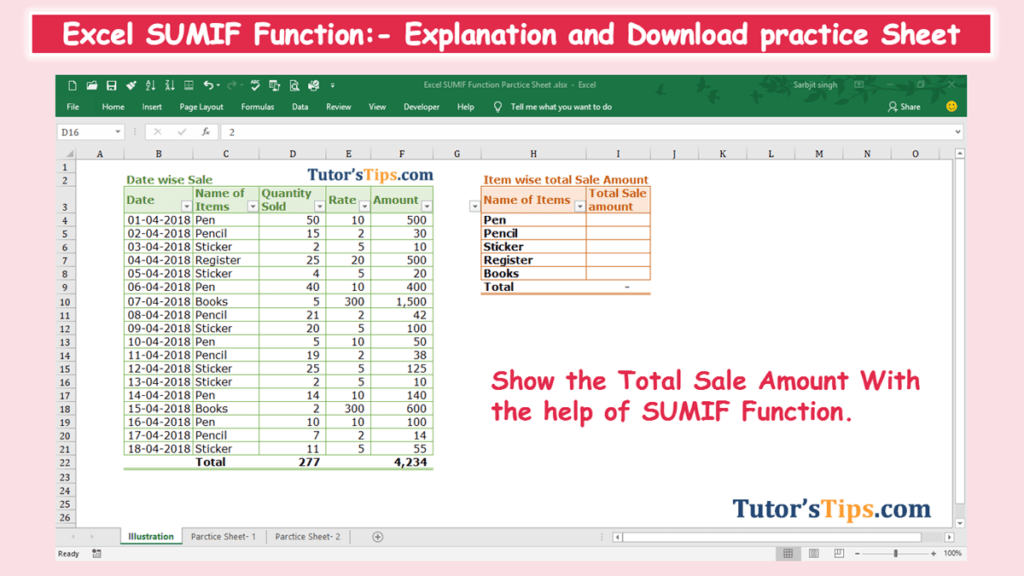The Excel SUMIF Function is used where we need to sum up the value on single criteria. It can be sum up the value of the cells based on a condition of any Text, number and logical text. It is also working on matching concepts like VLOOKUP and HLOOKUP but with a condition.

### The feature of “SUMIF” function: –

• With the help of SUMIF Function, we can retrieve the data if the condition is matched with the selected cells date.
• It helps in applying a particular condition on the data.

### The logical operator: –

The “SUMIF” function we can also use follow logical test on the selected data other than the Text and Number.

 Logical Test Meaning = Equal to <> Not Equal to > Greater than >= Greater than and Equal to < Less than <= Less than and equal to

### Explanation of Function:-

Now, We will explain the Arguments of the Function.

=SUMIF(range, criteria, [sum_range])

range: – Range is that column or row of selected cells on which we want to apply the condition or criteria.

criteria: –  This is a condition which we have to apply to the selected cells.

[sum_range]: – Sum range is that range of selected cells, which consist of the values which we want to total or summed up. This is optional because in the case where the range and sum_ range are the same. It means if we want to make a total of the value when a condition is cleared or matched from the other range of cells, not from the same column or row of the selected range on.

### Example of Function: –

I will show you, how to apply the whole function. (using optional also).

From the following table get the item-wise value of the total sale amount.

Solution:-

We will Apply the SUMif function and get the result. This is shown in the following steps: –

Step No. 1:-

We will write the “=SUMIF( ” in the column of the result.

Step No. 2

Now select the cells on which you want to apply the Condition or criteria.

In an example, we have to sum up the sale amount as per the name of the item. So, we have to apply a condition to the name of the items.

Step No. 3

Now select the Condition or criteria which we want to apply.

In an example, The name of items are given, we have to write the total amount in front of them, so we can select the criteria by selecting the cell of the given name.

Step No. 4

Now select the range of the cells of which the value we have to sum up.

In an example, The total amount of sale date wise give in the “F” column, so we select the cells of “F” column which have amount of sales.

Step No. 5: –

Now, Copy and paste the function in all remaining cell to get the result.